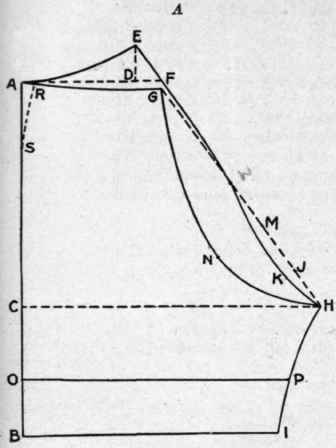Fold paper lengthwise

AB equals length to bend of knee (on fold). AG equals one-half AB plus two and three-quarter inches. AD equals one-quarter waist measure plus one-half inch. DE equals two inches.

AF equals one-quarter waist measure plus two inches. FG equals one-half inch. CH equals one-half hip measure.

BI equals one-half waist measure plus two and one-half inches. (This measure may vary according to individual preference. ) GH equals dotted line. HJ equals two and one-half inches.

JK equals three-quarter inch (K at right angles to J). HL equals nine inches.

HKLE equals curve of back. EM equals five inches. MN equals three inches (N at right angles to M). HNG equals curve of front. BO equals desired • depth of ruffle. IP equals desired depth of ruffle. PO equals line for bottom of drawers when ruffle is desired.

## To Cut Out Pattern

Trace lines AG,GNH,HI and IB. Open paper and cut on lines just traced; also AE, ELK, HI and IB.

Fulness at waist line may be fitted out in a dart over hip, as indicated by dotted line RS.

Fig. 61b shows pattern of drawers opened for cutting.Fig. 61. - Draft of pattern for straight drawers; A, draft; B, pattern opened out.

Circular Drawers (Fig. 62)

 Measures Required Standard Length from waist to bend of knee 24 inches Waist - around waist 24 inches Hip (over bone in hip, easy measure) 38 inches